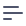# Getting Started with Cube.js Schema

Cube.js Schema is used to model raw data into meaningful business definitions and pre-aggregate and optimize results. Cube.js Schema is exposed as the querying API that allows end-users to query wide variety of analytical queries without modyfing Cube.js Schema itself.

Let’s use a users table with the following columns as an example:

idpayingcitycompany_name
1trueSan FranciscoPied Piper
2truePalo AltoRaviga
3trueRedwoodAviato
4falseMountain ViewBream-Hall
5falseSanta CruzHooli

We can start with a set of simple questions about users we want to answer:

• How many users do we have?
• How many paying users?
• What is the percentage of paying users out of the total?
• How many users, paying or not, are from different cities and companies?

We don’t need to write a SQL code for every question.
Cube.js Schema is all about building well-organized and reusable SQL.

## 1. Creating a Cube

In Cube.js, cubes are used to organize entities and connections between entities. Usually one cube is created for each table in the database, such as users, orders, products, etc. In the sql parameter of the cube we define a base table for this cube. In our case, the base table is simply our users table.

cube(Users, {
sql: SELECT * FROM users
});


## 2. Adding Measures and Dimensions

Once the base table is defined, the next step is to add measures and dimensions to the cube.

Measures are referred to as quantitative data, such as number of units sold, number of unique visits, profit, and so on.

Dimensions are referred to as categorical data, such as state, gender, product name, or units of time (e.g., day, week, month).

Let's go ahead and create our first measure and two dimensions.

cube(Users, {
sql: SELECT * FROM users,

measures: {
count: {
sql: id,
type: count
},
},

dimensions: {
city: {
sql: city,
type: string
},

companyName: {
sql: company_name,
type: string
}
}
});


Let's break down this code snippet by pieces. After defining the base table for the cube, we create a count measure in the Measures block. Type count and sql id means that when this measure will be requested via an API, Cube.js will generate and execute the following SQL:

SELECT count(id) from users;


When we apply a city dimension to the measure to see "Where are users based?" Cube.js will generate SQL with a GROUP BY clause:

SELECT city, count(id) from users GROUP BY 1;


You can add as many dimensions as you want to your query when you perform grouping.

## 3. Adding Filters to Measures

Now let's answer the next question – "How many paying users do we have?" To accomplish this, we will introduce measure filters:

cube(Users, {
measures: {
count: {
sql: id,
type: count
},

payingCount: {
sql: id,
type: count,
filters: [
{ sql: ${CUBE}.paying = 'true' } ] } } });  It is best practice to prefix references to table columns with the name of the cube or with the CUBE constant when referencing the current cube's column. That's it! Now we have the payingCount measure, which shows only our paying users. When this measure is requested, Cube.js will generate the following SQL: SELECT count( CASE WHEN (users.paying = 'true') THEN users.id END ) "users.paying_count" FROM users  Since the filters property is an array, you can apply as many filters as you like. payingCount can be used with dimensions the same way as a simple count. We can group payingCount by city and companyName simply by adding these dimensions alongside measures in the requested query. ## 4. Using Calculated Measures To answer "What is the percentage of paying users out of the total?" we need to calculate the paying users ratio, which is basically payingCount/count. Cube.js makes it extremely easy to perform this kind of calculation. Let's add a new measure to our cube called payingPercentage'. cube(Users, { measures: { count: { sql: id, type: count }, payingCount: { sql: id, type: count, filters: [ { sql: ${CUBE}.paying = 'true' }
]
},

payingPercentage: {
sql: 100.0 * ${payingCount} /${count},
type: number,
format: percent
}
}
});


Here we defined a calculated measure, payingPercentage, which is basically a division of payingCount by count. This example shows how you can reference measures inside other measure definitions. When you request the payingPercentage measure via an API, the following SQL will be generated:

SELECT
100.0 * count(
CASE WHEN (users.paying = 'true') THEN users.id END
) / count(users.id) "users.paying_percentage"
FROM users


Same as for other measures, payingPercentage` can be used with dimensions.

## 5. Next Steps